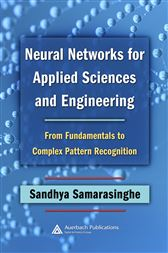Neural Networks for Applied Sciences and Engineering

From Fundamentals to Complex Pattern RecognitionUS\$57.95
In response to the exponentially increasing need to analyze vast amounts of data, Neural Networks for Applied Sciences and Engineering: From Fundamentals to Complex Pattern Recognition provides scientists with a simple but systematic introduction to neural networks.

Beginning with an introductory discussion on the role of neural networks in scientific data analysis, this book provides a solid foundation of basic neural network concepts. It contains an overview of neural network architectures for practical data analysis followed by extensive step-by-step coverage on linear networks, as well as, multi-layer perceptron for nonlinear prediction and classification explaining all stages of processing and model development illustrated through practical examples and case studies. Later chapters present an extensive coverage on Self Organizing Maps for nonlinear data clustering, recurrent networks for linear nonlinear time series forecasting, and other network types suitable for scientific data analysis.

With an easy to understand format using extensive graphical illustrations and multidisciplinary scientific context, this book fills the gap in the market for neural networks for multi-dimensional scientific data, and relates neural networks to statistics.

Features
§Explains neural networks in a multi-disciplinary context
§Uses extensive graphical illustrations to explain complex mathematical concepts for quick and easy understanding
?Examines in-depth neural networks for linear and nonlinear prediction, classification, clustering and forecasting
§Illustrates all stages of model development and interpretation of results, including data preprocessing, data dimensionality reduction, input selection, model development and validation, model uncertainty assessment, sensitivity analyses on inputs, errors and model parameters

Sandhya Samarasinghe obtained her MSc in Mechanical Engineering from Lumumba University in Russia and an MS and PhD in Engineering from Virginia Tech, USA. Her neural networks research focuses on theoretical understanding and advancements as well as practical implementations.

• CRC Press; April 2016
• ISBN: 9781420013061
• Edition: 1